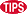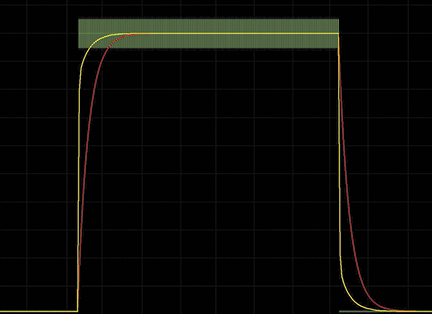Correcting For Error

# Zero Lag (Well, Almost)by John Ehlers and Ric Way

A little lag is a good thing. Here’s how you can remove a selected amount from an exponential moving average and use the filter in an effective trading strategy.

All smoothing filters and moving averages have lag. The lag is necessary because the smoothing is done using past data. Therefore, the averaging includes the effects of the data as of several bars ago. In this article we show you how to remove a selected amount of lag from an exponential moving average (Ema). Removing all the lag is not necessarily a good thing, because with no lag, the indicator would just track out the price you were filtering; the amount of lag removed is a tradeoff with the amount of smoothing you are willing to forgo. We show you the effects of lag removal in an indicator and then use the filter in an effective trading strategy.

The EMA
An exponential moving average (Ema) is computed by taking a fraction of the current price and adding to it the quantity (1 - fraction) times the previously computed value of the Ema. That fraction is called the “smoothing factor” and is commonly called α (alpha), and alpha is always less than 1. The equation for an Ema can be written as:

`EMA = α * Price + (1 - α) * EMA;`

where EMA is the value of the EMA one bar ago.Figure 1: zero lag ec (yellow) and ema (red) response to a step function. The SMA length is set to 12 and the gain limit is set to 50. Note that the EC indicator line has significantly faster rise and fall times than the EMA.

…Continued in the November issue of Technical Analysis of Stocks & Commodities